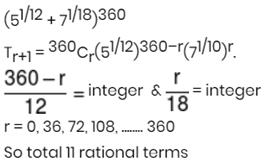Courses

# Maths Test 1 - Complex Number, Binomial Theorem, Permutation And Combination, Linear Inequality

## 25 Questions MCQ Test Mock Test Series for JEE Main & Advanced 2022 | Maths Test 1 - Complex Number, Binomial Theorem, Permutation And Combination, Linear Inequality

Description
This mock test of Maths Test 1 - Complex Number, Binomial Theorem, Permutation And Combination, Linear Inequality for JEE helps you for every JEE entrance exam. This contains 25 Multiple Choice Questions for JEE Maths Test 1 - Complex Number, Binomial Theorem, Permutation And Combination, Linear Inequality (mcq) to study with solutions a complete question bank. The solved questions answers in this Maths Test 1 - Complex Number, Binomial Theorem, Permutation And Combination, Linear Inequality quiz give you a good mix of easy questions and tough questions. JEE students definitely take this Maths Test 1 - Complex Number, Binomial Theorem, Permutation And Combination, Linear Inequality exercise for a better result in the exam. You can find other Maths Test 1 - Complex Number, Binomial Theorem, Permutation And Combination, Linear Inequality extra questions, long questions & short questions for JEE on EduRev as well by searching above.
QUESTION: 1

###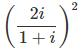is equal to

Solution: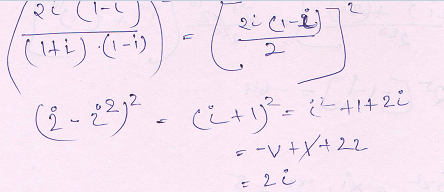QUESTION: 2

### Which of the following is true ?

Solution: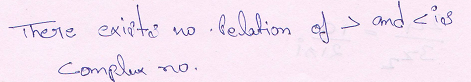QUESTION: 3

### If α is a complex number such that  α2+α+1 = 0 then α31  is equal to

Solution:
QUESTION: 4

Polar form of a complex number is

Solution:
QUESTION: 5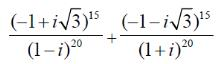is equal to

Solution: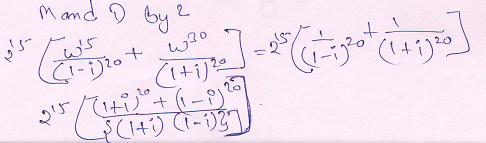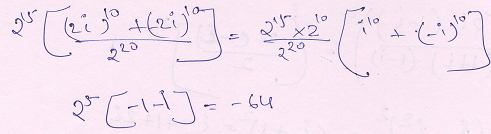QUESTION: 6

. Out of 7 consonants and 4 vowels, how many words of 3 consonants and 2 vowels can be formed?

Solution: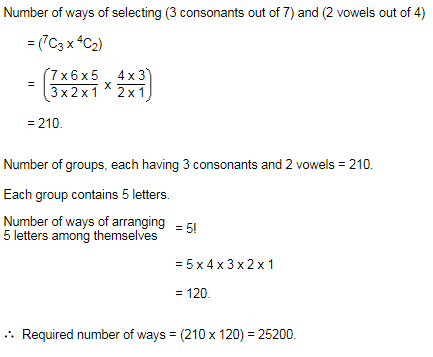QUESTION: 7

Number of non-zero integral solution of the equation  |1-i|x = 2 is

Solution: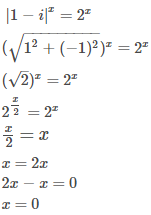QUESTION: 8

The number in the form z = a + bι where a, b ∈ R and i = √−1 is called a

Solution:
QUESTION: 9

In how many ways 7 men and 7 women can be seated around a round table such that no two women can sit together ?

Solution: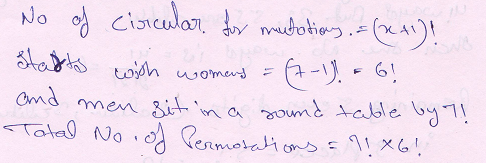QUESTION: 10

There are (n + 1) white and (n + 1) black balls, each set numbered 1 to n + 1. The number of ways the balls can be arranged in a row so that adjacent balls are of different colors, is

Solution: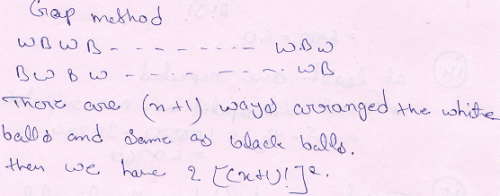QUESTION: 11

In z = a + bι, if i is replaced by −ι, then another complex number obtained is said to b

Solution:
QUESTION: 12

The sum of the digits at the unit place of all the numbers formed with the help of 3,4,5,6 taken all at a time is

Solution: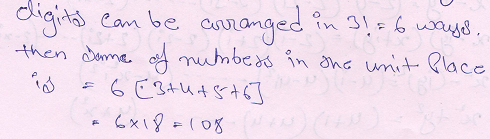QUESTION: 13

How many different nine digit numbers can be formed from the number 223355888 be rearranging its digits so that odd digits occupy even positions ?

Solution: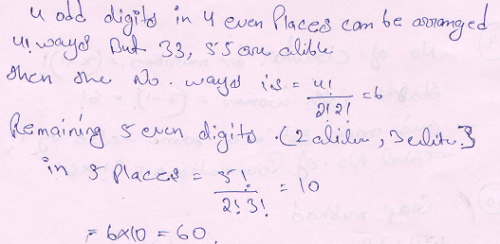QUESTION: 14

Ten different letters of an alphabet are given. Words with 5 letters are formed from these given letters. Then the number of words which have at least one letters repeated is

Solution: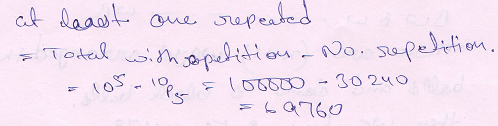QUESTION: 15
The straight lines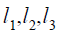are parallel and lie in the same plane. A total number of m points are taken on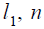points on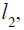k points on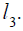The maximum number of triangles formed with vertices of these points are

Solution: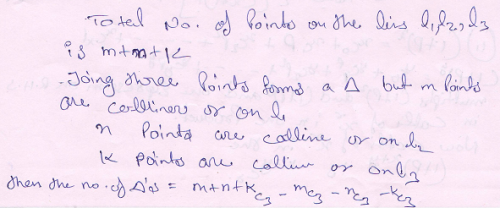QUESTION: 16

Six ‘X’s have to be placed in the squares of the figure given below such that each row contains at least one ‘X’. The number of ways in which this can be done is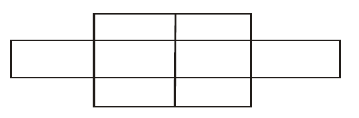Solution:

Number of ways of placed six X's in 8 squaresHere two squares of first and third row are empty

.. Required arrangements = 28 - 2 = 26

QUESTION: 17
If n is an integer between 0 and 21, then the minimum value of n!(21–n) ! is
Solution:QUESTION: 18

If the ratio of the 7th term from the beginning to the 7th term from the end in the expansion of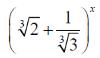is 1/6, then x is

Solution: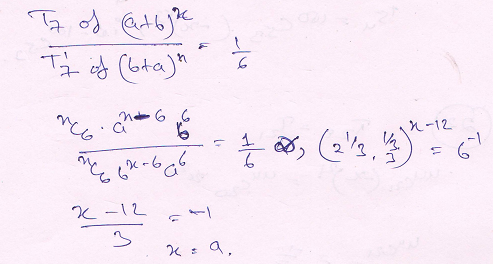QUESTION: 19
The middle term in the expansion of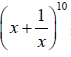is
Solution: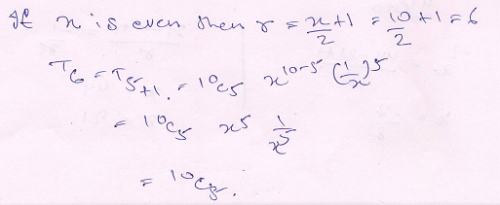QUESTION: 20

The value of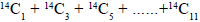is

Solution:

14C1 + 14C3 + 14C5 +.......14C11
For odd numbers : 2(n-1)
213 -14

*Answer can only contain numeric values
QUESTION: 21

By using 2, 4, 5, 7, 8, 9. How many three digit numbers are formed in form xyz when x < y and z < y (Repition not allowed) :-

Solution: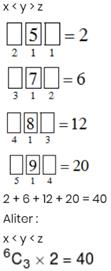*Answer can only contain numeric values
QUESTION: 22

If sum of the coefficient of the first, second and third terms of the expansion of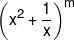is 46, then the coefficient of the term that does not contain x is :-

Solution: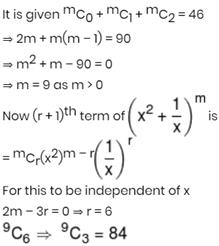*Answer can only contain numeric values
QUESTION: 23

If area of triangle formed by z, ωz and z + ωz is 16√3 square units (where ω is complex cube root of unity) the |z| is equal to :-

Solution: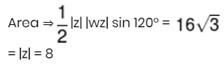*Answer can only contain numeric values
QUESTION: 24

If |z1| = 1, |z2| = 2, |z3| = 3 and |9z1 z2 + z2 z3 + 4z1 z3| = 12, then |z1 + z2 + z3| = ?

Solution: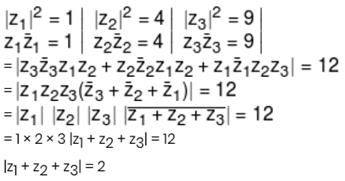*Answer can only contain numeric values
QUESTION: 25

Total number of rational terms in expansion of (51/12 + 71/18)360 is

Solution: